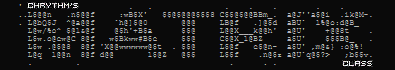# Matrix Class - Matrices Made Simple# Overview

Matrix math can get complex, and without a built-in class to deal with them, you may find yourself wrapped up with confusing layers of tables. This resource compresses matrices down into something simple and workable, while retaining table syntax and adding useful operations. Even without an understanding of the math behind the concept, this will enable you to deal with matrices like a pro.

### What is a matrix again?

A matrix is a grid of numbers organized into rows and columns. Doing this in code is the easy part. The hard part is dealing with all the different ways to deal with them, such as dot products.

This resource supports:
• Creating matrices
• Copying matrices
• Transposing matrices
• Checking if two matrices are equal
• Dot products for two matrices
• Dot product for a matrix and a vector
• Adding, subtracting, multiplying, and dividing
numbers, vectors, and other matrices
• Iterating a mutator function over each cell
• Negative matrices
• Improved printing (printing a matrix will show each cell)

Note: When I say vector, I really mean a table of numbers. I do this because we are usually limited to Vector2 and Vector1, but we often need much more than this.

### Usage

Example of creating a new Matrix and using table syntax:
``````local matrix = Matrix.new(
{3, 1, 1},
{5, 3, 2},
{6, 2, 9}
)

print(matrix) -- prints 3

matrix = 5 -- sets the third element in the second row to 5
``````

Example of using math operators:

``````local matrix1 = Matrix.new(
{3, 1, 1},
{5, 3, 2}
)

local matrix2 = Matrix.new(
{1, 3, 2},
{0, 5, 7}
)

print(matrix1 + matrix2) -- prints the sum of the matrices
print(matrix1 - matrix2) -- prints the difference of the matrices
print(matrix1 * matrix2) -- prints the product of the matrices
print(matrix1 / matrix2) -- prints the quotient of the matrices

-- you can also use vectors and numbers!

local vector = {1, 5, 3} -- notice that this is not a vector, but a table
-- we use tables to free ourselves from the limits of Vector3 and Vector2

print(matrix1 + vector) -- prints the matrix with the vector added to each row
print(matrix1 / 42) -- prints the matrix with each cell divided by the number 42
``````

Example of finding the dot product of two matrices:

``````local matrix1 = Matrix.new(
{3, 1, 1, 4},
{5, 3, 2, 1},
{6, 2, 9, 5}
)

local matrix2 = Matrix.new(
{4, 9},
{6, 8},
{9, 7},
{7, 6}
):Transpose()

print(matrix1:DotMatrix(matrix2))

--[[
Notice that we transpose matrix2 because a dot product requires
two matrices to have a common number of rows / columns. Here,
they share a common size of 4, where matrix1 has 4 columns, and
matrix2 has 4 rows.
]]
``````

You can also find these examples inside the module.

### Why?

The closest you can get to matrices through code is by using nested tables. Even then, you need to know how to operate on them in order to get desired results. This requires a strong understanding of vector math, and here in the programming world, we like to let the computer do the heavy lifting.

More specifically, the motivation behind this resource was to shrink neural network code.

### Notes

• The math operators and operations do not modify the matrices, they return copies with modifications. For example, adding a number to a matrix will return a modified copy of that matrix with the number added. You should overwrite the original with the copy if you wish to modify it.

• If you want a copy of a matrix, you can call matrix:Clone()

• The math operators (such as +, -, *, /) actually just call methods depending on what is being operated on. For example, if you add a vector to a matrix, it will figure out what method to call. At the same time, you can add numbers and other matrices the same way.